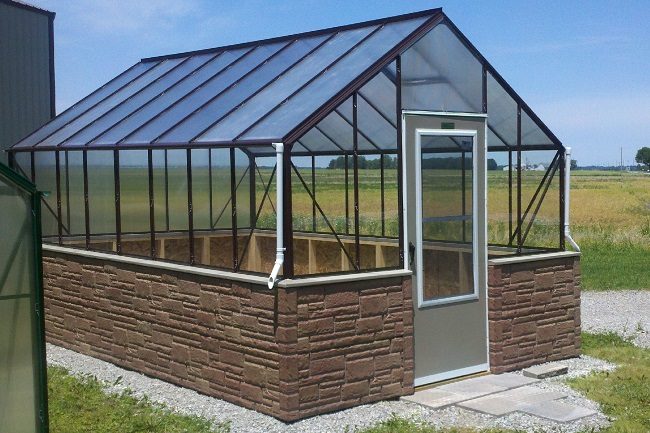# How to Calculate the Number of Polycarbonate Sheets and Profiles Needed to Build a GreenhouseI get a lot of questions about how to figure the polycarbonate sheets and profiles needed to build a greenhouse. The best way to handle this is to break the project down into sections. To do this figure each section and then add them all together to get your final bill of material. This article includes an example of how I would figure a 12’ x 24’ greenhouse with 6′ sidewalls and an 8′ peak height. The roof panels will be 6′ 6″. This gives the greenhouse slightly less than a 3″ overhang on the roof panels.

###### The Profiles are Available in the These Lengths

H profile (connecting sheets side by side) 12′ or 24′

R profile (ridge profile) 12′ or 24′

U profile (this caps off the channels of the sheets, used on the tops and bottoms of the sheets) 12′

F profile (for corners or flashing) 12′ or 24′

###### Roof

It is easiest to figure one side and then multiply it by 2. Each side would have 6 sheets at a 4′ width by 6′ 6″. When you are figuring the H’s (sheet connectors side by side) simply subtract 1 from the total number of sheets on each side. For this example, you would need 5 H’s at 7′. You would want to order 5 H’s at 12′, as this is the length they are available in. You do not want to use a “patched together” H on your roof (or at any time really).

If you are using a polycarbonate R or ridge, you would need one piece at 24′. To figure the U’s you would need to figure the linear foot total for the bottom and tops of the sheets. The exception to this is if you are using a ridge. The ridge is already serving the same purpose as the U at the top of the sheet. Since we are using the ridge we need 24′ of U, or 2 pieces at 12′. Multiply this by 2 and you are done.

###### Side Walls

Figure one side and then multiply it by 2. Each side would have 6 sheets at 4′ x 6′. To figure the H’s (sheet connectors side by side) simply subtract 1 from the total number of sheets on each side. For this example, 5 H’s at 7′ are needed but you would order 5 H’s at 12′, as this is the length they are available in.

To figure the U’s figure the linear foot total for the bottom and tops of the sheets. For 6 sheets 4′ wide you would need 24′ at the top and 24′ at the bottom for a total of 4 – 12′ U profiles. Multiply this by 2 and you are done.

The corners also need to be calculated and in this case 4 F profiles at 6′ are needed. I would order one 24′ F profile for the entire job.

###### Gable Ends

Calculate one side and then multiply by 2. It is best to have one sheet the entire length from the ridge to the ground. An angle will need to be cut on site. For this case order 3 sheets at 4′ x 8′.

To figure the H’s (sheet connectors side by side) simply subtract 1 from the total number of sheets on each side. For this example 2 H’s at 8′ are needed. Order 2 H’s at 12′, as this is the length they are available in.

To figure the U’s calculate the linear foot total for the bottom and tops of the sheets. For 3 sheets at 4′ you would need one 12′ U for the bottom. You also need a U for the gables. The roof panels are 6′ 6″, so this would be the approximate length of U needed to cover the gable ends. Order 2 of the 12′ U’s for the gables. This end would need a total of 3 – 12′ U’s. There will be a door on one end so this will need to be adjusted for the other end. To do this cut down on your sheets and also be sure to add enough U to frame out your door where you have cut the polycarbonate sheets.

Tammy Wylie has been selling and installing greenhouses since 1993 and is the owner of Advance Greenhouses. You can visit her website at AdvanceGreenhouses.com.